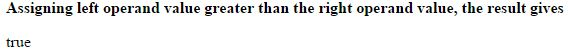# Greater than (>) operator in JavaScript

### Greater than (>)

Greater than operator is an comparison operator which is used to check the value of left operand is greater than the value of the right operand.

If the left operand value is greater thean the right operand value it returns 'true'.

The symbolic representation of greater than is `>`.

### Left operand is greater than right operand

```<b>Assigning left operand value greater than the right operand value, the result gives</b>
<p id="myId"></p>

<script>
var a = 10;
var b = 5;
var c = (a > b);
document.getElementById("myId").innerHTML = c;
</script>
```

In the above code snippet we assigned greater value to the left operand and lesser value to the right operand, we used greater than `>` operator, so the result gives 'true'.

OUTPUT### Left operand is lesser than right operand

```<b>Assigning left operand value lesser than the right operand value, the result gives</b>
<p id="myId"></p>

<script>
var a = 5;
var b = 10;
var c = (a > b);
document.getElementById("myId").innerHTML = c;
</script>```

Greater than

In the above code snippet we assigned lesser value to the left operand and greater value to the right operand, so the result gives 'false'.

OUTPUTViews: 2886 | Post Order: 171